1. /
2. CBSE
3. /
4. Class 09
5. /
6. Mathematics
7. /
8. NCERT Solutions for Class...

# NCERT Solutions for Class 9 Maths Exercise 13.1### myCBSEguide App

Download the app to get CBSE Sample Papers 2023-24, NCERT Solutions (Revised), Most Important Questions, Previous Year Question Bank, Mock Tests, and Detailed Notes.

NCERT solutions for Class 9 Maths Surface Areas and Volumes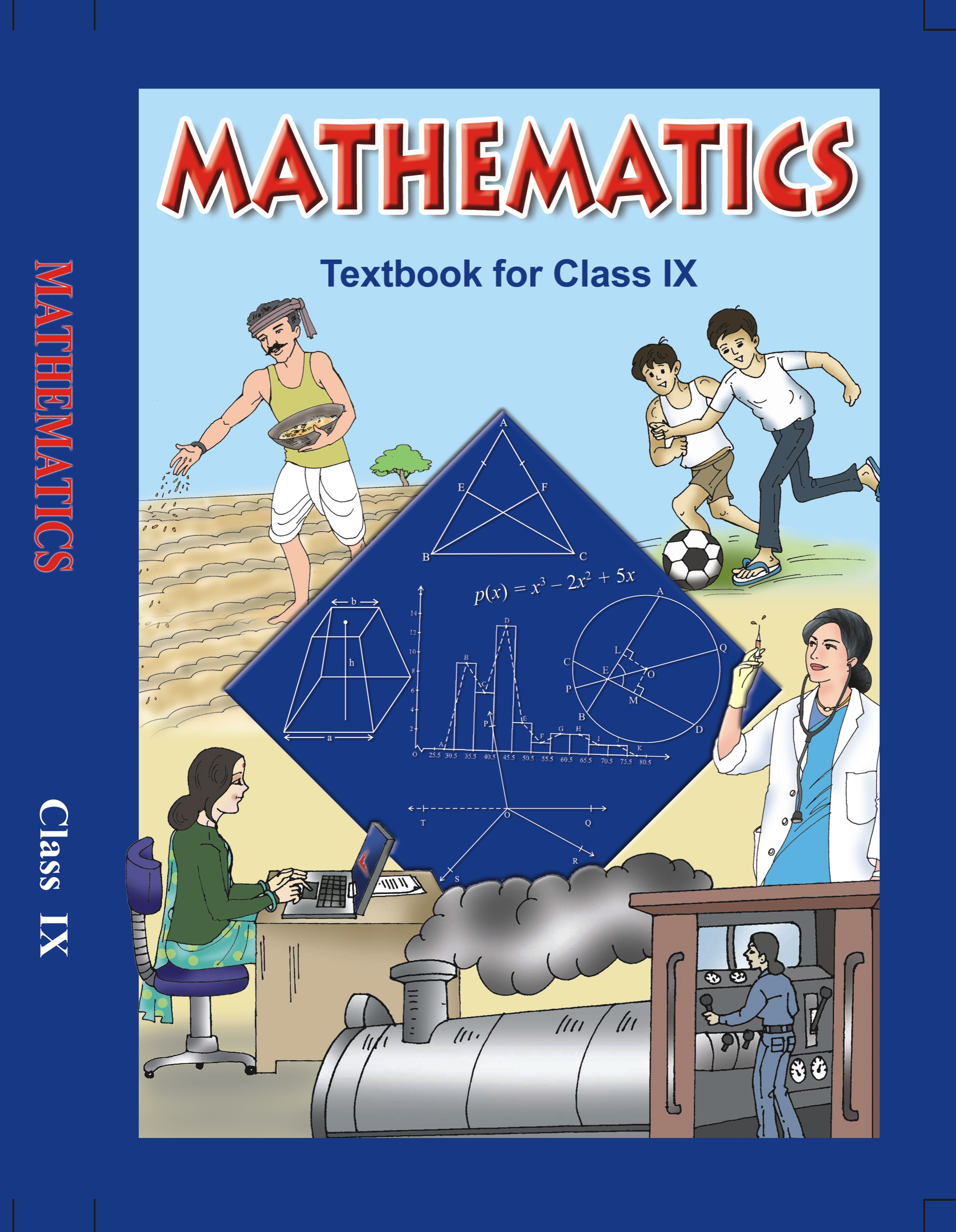## NCERT Solutions for Class 9 Mathematics Surface Areas and Volumes

### 1. A plastic box 1.5 m long, 1.25 m wide and 65 cm deep is to be made. It is to be open at the top. Ignoring the thickness of the plastic sheet, determine:

(i) The area of the sheet required for making the box.

(ii) The cost of sheet for it, if a sheet measuring 1m2 cost Rs.20.

Ans. (i) Given: Length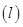= 1.5 m, Breadth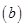= 1.25 m and Depth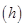= 65 cm = 0.65 m

Area of the sheet required for making the box open at the top =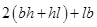=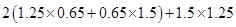=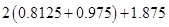=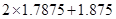= 3.575 + 1.875 = 5.45 m2

(ii) Since, Cost of 1 m2 sheet = Rs. 20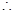Cost of 5.45 m2 sheet = 20 x 5.45 = Rs. 109

### 2. The length, breadth and height of a room are 5 m, 4 m and 3 m respectively. Find the cost of white washing the walls of the room and the ceiling at the rate of Rs. 7.50 per m2.

Ans. Given: Length= 5 m, Breadth= 4 m and Height= 3 m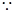Area of the four walls = Lateral surface area =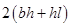=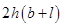= 2 x 3 (4 + 5)

= 2 x 9 x 3 = 54 m2

Area of ceiling =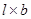= 5 x 4 = 20 m2Total area of walls and ceiling of the room = 54 + 20 = 74 m2

Now Cost of white washing for 1 m2 = Rs. 7.50Cost of white washing for 74 m2 = 74 x 7.50 = Rs. 555

NCERT Solutions for Class 9 Maths Exercise 13.1

### 3. The floor of a rectangular hall has a perimeter 250 m. If the cost of painting the four walls at the rate of Rs. 10 per m2 is Rs. 15000, find the height of the hall.

Ans. Given: Perimeter of rectangular wall

=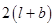= 250 m ……….(i)

Now Area of the four walls of the room

=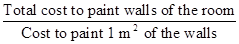=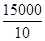= 1500 m2 ……….(ii)Area of the four walls = Lateral surface area

=== 1500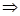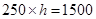[using eq. (i) and (ii)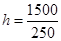= 6 m

Hence required height of the hall is 6 m.

NCERT Solutions for Class 9 Maths Exercise 13.1

### 4. The paint in a certain container is sufficient to paint an area equal to 9.375 m2. How many bricks of dimensions 22.5 cm x 10 cm x 7.5 cm can be painted out of this container?

Ans. Given: Length of the brick= 22.5 cm, Breadth= 10 cm and Height= 7.5 mSurface area of the brick

=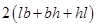= 2 (22.5 x 10 + 10 x 7.5 + 7.5 x 22.5)

= 2 (225 + 75 + 468.75)

= 937.5 cm2

= 0.09375 m2 [1 cm = 0.01 m]

Now No. of bricks to be painted

=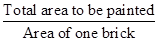=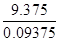= 100

Hence 100 bricks can be painted.

NCERT Solutions for Class 9 Maths Exercise 13.1

### 5. A cubical box has each edge 10 cm and a cuboidal box is 10 cm wide, 12.5 cm long and 8 cm high.

(i) Which box has the greater lateral surface area and by how much?

(ii) Which box has the smaller total surface area and how much?

Ans. (i) Lateral surface area of a cube = 4 (side)2 = 4 x (10)2 = 400 cm2

Lateral surface area of a cuboid =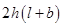= 2 x 8 (12.5 + 10) = 16 x 22.5 = 360 cm2Lateral surface area of cubical box is greater by (400 – 360) = 40 cm2

(ii) Total surface area of a cube = 6 (side)2

= 6 x (10)2 = 600 cm2

Total surface area of cuboid == 2 (12.5 x 10 + 10 x 8 + 8 x 12.5)

= 2 (125 + 80 + 100)

= 2 x 305 = 610 cm2Total surface area of cuboid box is greater by (610 – 600) = 10 cm2

NCERT Solutions for Class 9 Maths Exercise 13.1

### 6. A small indoor green house (herbarium) is made entirely of glass panes (including base) held together with tape. It is 30 cm long, 25 cm wide and 25 cm high.

(i) What is the surface area of the glass?

(ii) How much of tape is needed for all the 12 edges?

Ans. (i) Given: Length of glass herbarium= 30 cm,

Breadth= 25 cm and Height= 25 m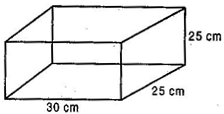Total surface area of the glass

== 2 (30 x 25 + 25 x 25 + 25 x 30)

= 2 (750 + 625 + 750)

= 2 x 2125 = 4250 cm2

Hence 4250 cm2 of the glass is required to make a herbarium.

(ii) Tape is used at 12 edges.Tape is used at 4 lengths, 4 breadths and 4 heights.Total length of the tape =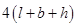= 2 (30 + 25 + 25) = 320 cm

Hence 320 cm of the tape if needed to fix 12 edges of herbarium.

NCERT Solutions for Class 9 Maths Exercise 13.1

### 7. Shanti Sweets Stall was placing an order for making cardboard boxes for packing their sweets. Two sizes of boxes were required. The bigger of dimensions 25 cm by 20 cm by 5 cm and the smaller of dimensions 15 cm by 12 cm by 5 cm. 5% of the total surface area is required extra, for all the overlaps. If the cost of the card board is Rs. 4 for 1000 cm2, find the cost of cardboard required for supplying 250 boxes of each kind.

Ans. Given: Length of bigger cardboard box (L) = 25 cm

Breadth (B) = 20 cm and Height (H) = 5cm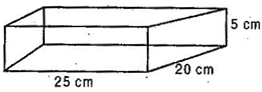Total surface area of bigger cardboard box

= 2 (LB + BH + HL)

= 2 (25 x 20 + 20 x 5 + 5 x 25)

= 2 (500 + 100 + 125)

= 1450 cm2

5% extra surface of total surface area is required for all the overlaps.5% of 1450 =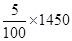= 72.5 cm2

Now, total surface area of bigger cardboard box with extra overlaps

= 1450 + 72.5 = 1522.5 cm2Total surface area with extra overlaps of 250 such boxes

= 250 x 1522.5 = 380625 cm2

Since, Cost of the cardboard for 1000 cm2 = Rs. 4Cost of the cardboard for 1cm2 = Rs.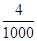Cost of the cardboard for 380625 cm2

= Rs.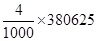= Rs. 1522.50

Now length of the smaller box= 15 cm,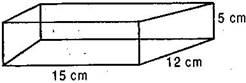Breadth= 12 cm and Height= 5 cm

Total surface area of the smaller cardboard box

== 2 (15 x 12 + 12 x 5 + 5 x 15)

= 2 (180 + 60 +75) = 2 x 315 = 630 cm2

5% of extra surface of total surface area is required for all the overlaps.5% of 630 =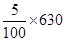= 31.5 cm2

Total surface area with extra overlaps = 630 + 31.5 = 661.5 cm2

Now Total surface area with extra overlaps of 250 such smaller boxes

= 661.5 x 250 = 165375 cm2

Cost of the cardboard for 1000 cm2 = Rs. 4

Cost of the cardboard for 1cm2 = Rs.Cost of the cardboard for 165375 cm2 = Rs.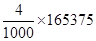= Rs. 661.50Total cost of the cardboard required for supplying 250 boxes of each kind

= Total cost of bigger boxes + Total cost of smaller boxes

= Rs. 1522.50 + Rs. 661.50

= Rs. 2184

NCERT Solutions for Class 9 Maths Exercise 13.1

### 8. Parveen wanted to make a temporary shelter for her car, by making a box-like structure with tarpaulin that covers all the four sides and the top of the car (with the front face as a flap which can be rolled up). Assuming that the stitching margins are very small and therefore negligible, how much tarpaulin would be required to make the shelter of height 2.5 m with base simensions 4 m x 3 m?

Ans. Given: Length of base= 4 m, Breadth= 3 m and Height= 2.5 m

Ans. Tarpaulin required to make shelter

= Surface area of 4 walls + Area of roof

=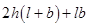= 2 (4 + 3) 2.5 + 4 x 3

= 35 + 12 = 47 m2

Hence 47 m2 of the tarpaulin is required to make the shelter for the car.

## NCERT Solutions for Class 9 Maths Exercise 13.1

NCERT Solutions for Class 9 Maths PDF (Download) Free from myCBSEguide app and myCBSEguide website. Ncert solution class 9 Maths includes text book solutions from Mathematics Book. NCERT Solutions for CBSE Class 9 Maths have total 15 chapters. 9 Maths NCERT Solutions in PDF for free Download on our website. Ncert Maths class 9 solutions PDF and Maths ncert class 9 PDF solutions with latest modifications and as per the latest CBSE syllabus are only available in myCBSEguide.

## CBSE app for Class 9

To download NCERT Solutions for Class 9 Maths, Computer Science, Home Science,Hindi ,English, Social Science do check myCBSEguide app or website. myCBSEguide provides sample papers with solution, test papers for chapter-wise practice, NCERT solutions, NCERT Exemplar solutions, quick revision notes for ready reference, CBSE guess papers and CBSE important question papers. Sample Paper all are made available through the best app for CBSE students and myCBSEguide website.Test Generator

Create question paper PDF and online tests with your own name & logo in minutes.myCBSEguide

Question Bank, Mock Tests, Exam Papers, NCERT Solutions, Sample Papers, Notes

### 12 thoughts on “NCERT Solutions for Class 9 Maths Exercise 13.1”

1. Thanks a lot……………………………………

2. Nice

???????

???????

5. thanks it help me in understanding more clear

6. so good

7. Good to help others with this answers it helped me a lot

8. Good for student

9. Thanks so much

10. thnx for this

11. Thankful app

12. very good app